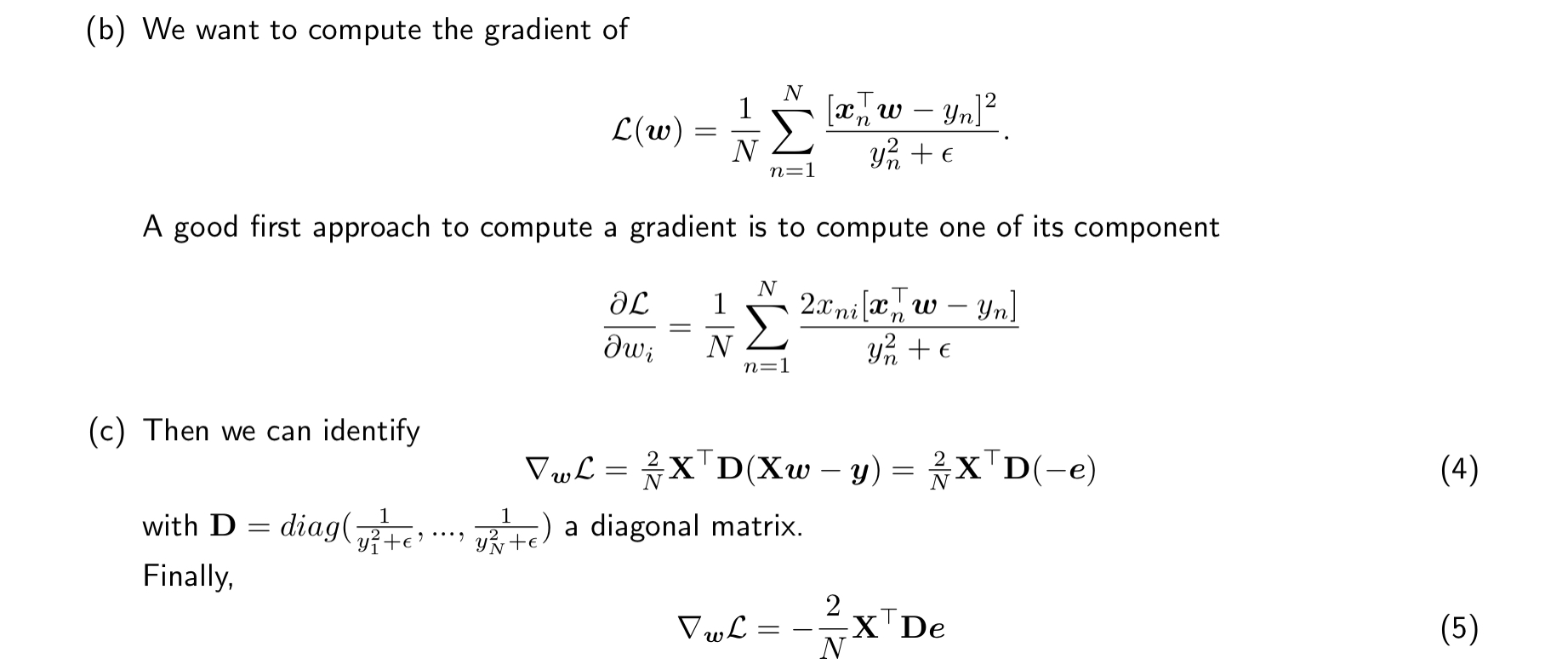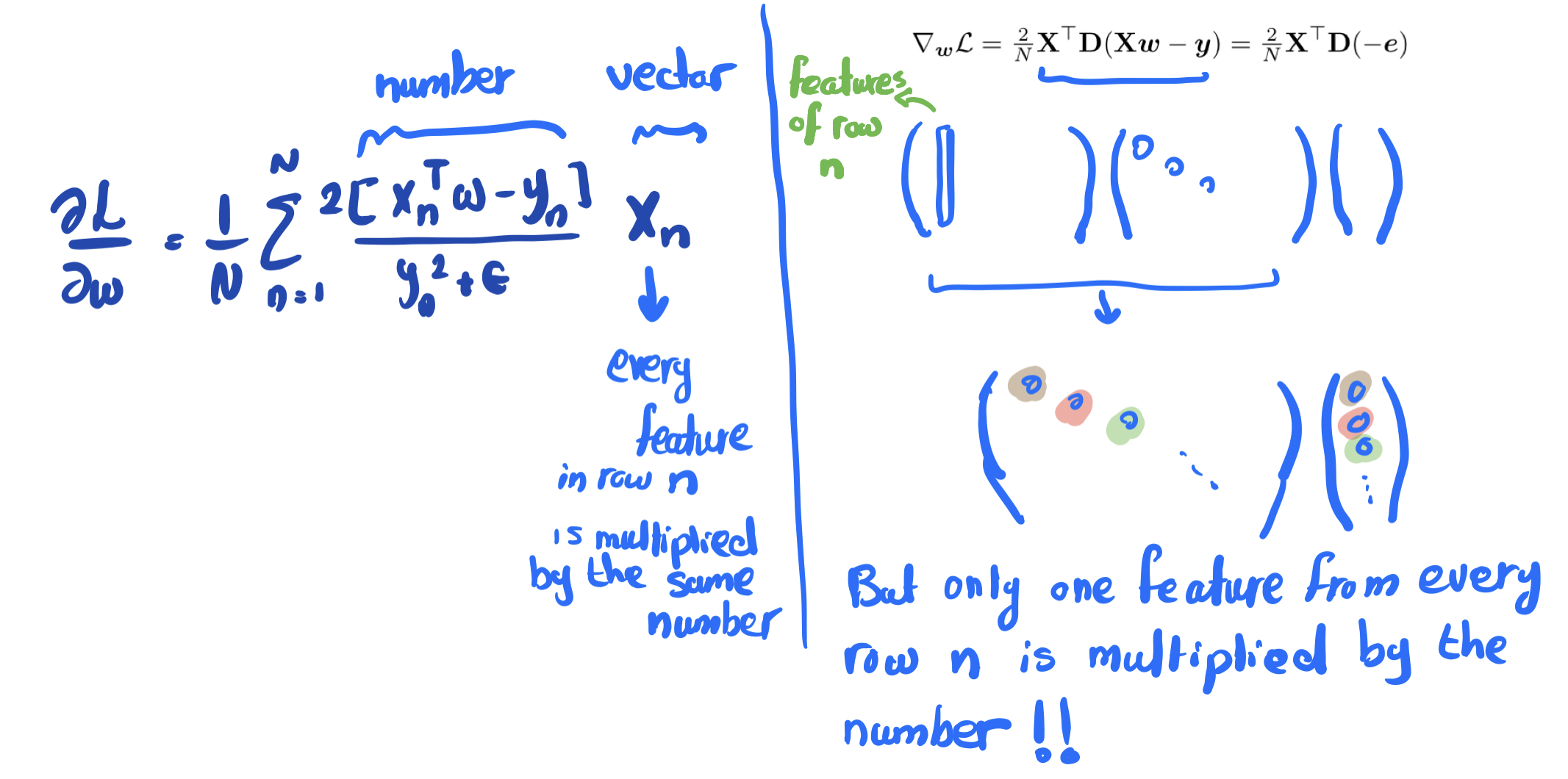### Problem set 3, compute the gradient for a cost function

Hello,

I don’t see that the provided solution gives us the behavior that we would expect. When we introduce the diagonal matrix many ‘features’ in X will be lost because they are multiplied with 0. And this is not what the indices notation shows.

How can I see that the solution (eq. 5) makes sense?And for the same question part D. I don’t really buy the answer because if we have y = 100 and y_hat = 1, we will have very small loss and the function will not be sensitive to outliers (and it will not really take the relative error into account as claimed in the exercise)Page 1 of 1